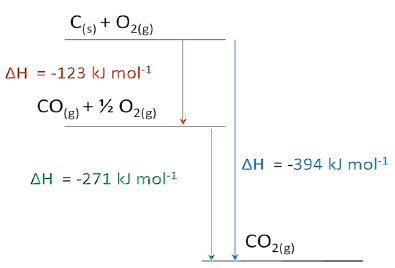Chemistry

# Calculating the Enthalpy Change of a Reaction

The simplest way to apply Hess’s Law is to remember that the enthalpy change of any reaction is equal to the total formation energy of the products minus that of the reactants as shown below:

ΔH° = ∑ nΔHf (products) – ∑ mΔHf (reactants)

Where ∑ is the mathematical symbol meaning “the sum of”, and m and n are the coefficients of the substances in the chemical equation.

Enthalpy diagram The corresponding enthalpy diagram (also called “pictorial method”) for the three thermo-chemical equations discussed above is shown in the Figure below: